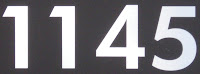## Tuesday, July 20, 2010

### 1145

1145 = 5 x 229.

1145 has two representations as a sum of two squares: 1145 = 112 + 322 = 192 + 282.

1145 is the hypotenuse of two Pythagorean triples: 11452 = 4232 + 10642 = 7042 + 9032.

1145, 1147 (31 x 37), and 1149 (3 x 383) are each semiprimes, the product of two primes.Halley's Comet appeared in the skies in the year 1145.

Source: WolframAlpha

#### 1 comment:Anonymous said...

Other representations of number 1145:
1145 =11^2+4^5=11^2+2^10
As Pythagorean triples
1145^2+26208^2=26233^2
1145^2+655512^2=655513^2
As the sum of a square plus a prime number
1145=4^2+1129=18^2+821=22^2+661=24^2+569
As a sum or difference of plus or minus power a prime number
1145=6^3+929=2^4+1129
1145=6^4-151=34^2-11
As the sum of a square with a cousin by a power
1145=3^2+71*4^2=30^2+5*7^2 =13^2+61*4^2=29^2+19*4^2
1145=7^2+137*2^3=17^2+107*2^3=21^2 +11*2^6=27^2+13*2^5=31^2+23*2^3=33^2+7*2^3
As more power sum minus the product of a cousin with other Power
1145=9^3+13*2^5=3^6+13*2^5=2^7+113*3^2
Rafael Parra de Barcelona